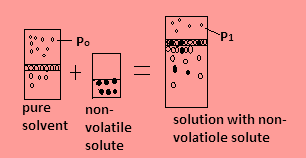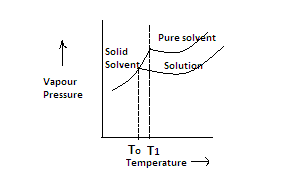QuestionAnswers

# In the depression of freezing point experiment, it is found that-A) The vapour pressure of the solution is less than that of pure solvent.B) The vapour pressure of solution is more than that of pure solvent.C) Only solute molecules solidify at the freezing point.D) Only solvent molecules solidify at the freezing point.Hint: When non-volatile solute is added in solvent, a solution is formed. The vapour pressure of the solution decreases and the substance present in larger amounts solidifies when vapour pressure of solid becomes equal to the vapour pressure of liquid.

-When a non-volatile solute is added in a pure solvent, some solute molecules occupy the surface area along with molecules of pure solvent and the pure solvent’s tendency to go into vapour phase decreases due to which the vapour pressure of solution decreases. This means that the vapor pressure of solid and liquid solvent becomes equal at lower temperature. This is called the depression in the freezing point.
-Let us take example –Suppose in the closed container, water is the pure solvent in the figure and its freezing point is ${0^ \circ }{\text{C}}$ and water’s vapour pressure is suppose ${{\text{P}}_0}$ as evaporation at the surface of the water continues then-
1. When non-volatile solute is added in the water, the solution formed has vapor pressure ${{\text{P}}_1}$ which is less than the vapour pressure of the solvent as the number of particles in the solution increases due to solute and they occupy the surface area but they have less tendency to forms vapours.
2. The freezing point of solution becomes $- {1^ \circ }{\text{C}}$ or less. This means the freezing point decreases when non-volatile solute is added. Since the molecules of solvent are more than that of solute so the molecules of solvent solidify at freezing point.

Hence, correct options are A and D.

In the given graph, relation between vapour pressure and temperature is given for depression of freezing point-1. The pure solvent has more vapour pressure than the solution due to presence of non-volatile solute so its curve is drawn higher than the curve of solution. Now the vapors of solid solvent form a steep curve.
2. When the temperature at which the vapor pressure of solid solvent and liquid solvent equal becomes then it is called freezing point. This temperature is given by ${{\text{T}}_0}$.
3. When we draw the curve of solution to meet the curve of solid solvent we see that their vapor pressure becomes equal at lower temperature which is represented by ${{\text{T}}_1}$ .
4.The difference between these two temperatures is called depression in freezing point.
It is given as-
$\Delta {\text{T}} = {{\text{T}}_{\text{0}}}{\text{ - }}{{\text{T}}_{\text{1}}}$

Note:
Depression of freezing point is also given as-
$\Delta {\text{T = }}{{\text{K}}_f}{\text{m}}$ where m is the molality and ${{\text{K}}_f}$ is the cryoscopic constant which depends on the properties of solvent and solute. Molality is the number of moles of solutes dissolved in one kilogram solvent.
View Notes
Inbreeding DepressionMillikan's Oil Drop ExperimentBoiling Point FormulaElectric Potential - Point ChargeElectric Field Due to Point ChargeNCERT Book for Class 12 Chemistry PDF in HindiWater Clock ExperimentMagnetic and Electric Force on a Point ChargeLava Lamp ExperimentSignificance of Genetics in the Process of EvolutionImportant Questions for CBSE Class 12 Chemistry Chapter 16 - Chemistry in Everyday lifeImportant Questions for CBSE Class 12 Chemistry Chapter 1 - The Solid StateImportant Questions for CBSE Class 12 ChemistryImportant Questions for CBSE Class 12 Chemistry Chapter 5 - Surface ChemistryImportant Questions for CBSE Class 12 Chemistry Chapter 7 - The p-Block ElementsNCERT Books Free Download for Class 12 Chemistry Chapter 16 - Chemistry in Everyday lifeImportant Questions for CBSE Class 12 Chemistry Chapter 8 - The d and f Block ElementsImportant Questions for CBSE Class 11 Chemistry Chapter 3 - Classification of Elements and Periodicity in PropertiesImportant Questions for CBSE Class 12 Chemistry Chapter 6 - General Principles and Processes of Isolation of ElementsImportant Questions for CBSE Class 12 Chemistry Chapter 3 - ElectrochemistryChemistry Question Paper for CBSE Class 12CBSE Class 12 Chemistry Question Paper 2020Chemistry Question Paper for CBSE Class 12 - 2013Chemistry Question Paper for CBSE Class 12 - 2015CBSE Class 12 Chemistry Question Paper 2019 - Free PDFCBSE Class 12 Chemistry Question Paper 2017 - Free PDFCBSE Class 12 Chemistry Question Paper 2018 - Free PDFPrevious Year Question Paper for CBSE Class 12 Chemistry - 2014Chemistry Question Paper for CBSE Class 12 - 2016 Set 1 EChemistry Question Paper for CBSE Class 12 - 2016 Set 1 SNCERT Solutions for Class 12 Chemistry Chapter 1 The Solid State in HindiNCERT Solutions for Class 12 Chemistry Chapter 16 Chemistry in Everyday life in HindiNCERT Solutions for Class 12 Chemistry in HindiNCERT Solutions for Class 12 Chemistry Chapter 5 Surface Chemistry in HindiNCERT Solutions for Class 12 Chemistry Chapter 7 The p-Block Elements In HindiNCERT Solutions for Class 12 Chemistry Chapter 12 Aldehydes, Ketones and Carboxylic Acids in HindiNCERT Solutions for Class 12 Chemistry Chapter 16NCERT Solutions for Class 12 Chemistry Chapter 8 The d and f Block Elements in HindiNCERT Solutions for Class 12 Chemistry Chapter 6 General Principles and Processes of Isolation of Elements in HindiNCERT Solutions for Class 11 Chemistry Chapter 1 Some Basic Concepts of Chemistry in Hindi